View or download the JEE Main 2021 July 20 shift 2 Physics question paper with solutions from this page. Each of the questions asked from the Physics section has been analyzed and solved by our expert teachers. Thus, the solutions have been prepared in a detailed manner. With our approach, we are addressing the need of students to find the right answer to each of the questions asked in JEE Main 2021 session 3 Paper.

The questions and solutions document which is available in a PDF format can be downloaded instantly for offline use. JEE aspirants can use this study resource to prepare effectively for the JEE exam.

July 20 Shift 2 – Physics Question Paper and Solutions

Question 1: If the kinetic energy of a particle becomes four times, then % change in momentum will be:

a. 200
b. 100
c. 150
d. 50

K.E. ⇒ K = P2 / 2m

P ∝ √K

$$\begin{array}{l}\frac{P_{2}}{P_{1}} = \sqrt{\frac{K_{2}}{K_{1}}} \Rightarrow \frac{P_{2}}{P_{1}} = \sqrt{\frac{4K}{K}}\end{array}$$

$$\begin{array}{l}\frac{P_{2}}{P_{1}} = 2\end{array}$$

$$\begin{array}{l}\frac{P_{2} – P_{1}}{P_{1}}% = \frac{P_{2}}{P_{1}-1}\times 100 = (2-1)\times 100 = 100\end{array}$$

$$\begin{array}{l}\frac{\Delta P}{P_{1}}% = 100%\end{array}$$

Question 2: A RLC circuit is in its resonance condition. Its circuit components have value R = 5Ω, L = 2H, C = 0.5mF, V= 250V. Then find the power in the circuit.

a. 6 kW
b. 10 kW
c. 12 kW
d. 12.5 kW

As the circuit is in resonance. Thus,

XL = XC

∴ Z = R so, irms = V / Z = V / R

$$\begin{array}{l}P = i_{rms}^{2}R\end{array}$$
$$\begin{array}{l}P = \frac{V^{2}}{R} = \frac{250\times 250}{5} = 12500\frac{J}{S} = 12.5\: kW\end{array}$$

Question 3: A satellite is revolving around a planet in an orbit of radius R. Suddenly radius of orbit becomes 1.02 R, then what will be the percentage change in its time period of revolution?

a. 2%
b. 3%
c. 5%
d. 7%

$$\begin{array}{l}T \alpha R^{\frac{3}{2}}\end{array}$$
$$\begin{array}{l}T = kR^{\frac{3}{2}}\end{array}$$
$$\begin{array}{l}\frac{\Delta T}{T} = \frac{3}{2}\frac{\Delta R}{R} = 3%\end{array}$$
%

Question 4: A person walks up a stationary escalator in the time 𝑡1. If he remains stationary on the escalator, then it can take him up in time 𝑡2. Determine the time it would take to walk upon the moving escalator?

a.

$$\begin{array}{l}\frac{t_{1}t_{2}}{t_{1}+t_{2}}\end{array}$$

b.
$$\begin{array}{l}\frac{t_{1}t_{2}}{t_{1}-t_{2}}\end{array}$$

c.
$$\begin{array}{l}\frac{2t_{1}t_{2}}{t_{1}+t_{2}}\end{array}$$

d.
$$\begin{array}{l}\frac{2t_{1}t_{2}}{t_{1}-t_{2}}\end{array}$$

Suppose length of escalator = L

Speed of man w.r.t escalator L / t1

Speed of escalator = L / t2

Speed of man w.r.t ground when escalator is moving = L / t1 + L / t2

Time taken by the man to walk on the moving escalator =

$$\begin{array}{l}\frac{L}{\frac{L}{t_{1}}+\frac{L}{t_{2}}} = \frac{t_{1}t_{2}}{t_{1}+t_{2}}\end{array}$$

Question 5: For the given graph between decay rate and time find half-life (where R = decay rate).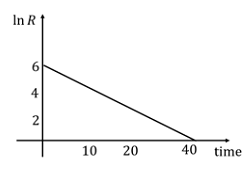a. 10 / 3 ln⁡2
b. 20 / 3 ln⁡2
c. 3 / 20 ln⁡2
d. 3 / 10 ln⁡2

$$\begin{array}{l}R = R_{o}e^{-\lambda t}\end{array}$$

ln R = ln ⁡Ro – λt

Slope = -λ = – 6 / 40

λ = 3 / 20

$$\begin{array}{l}t_{1/2} = \frac{ln2}{\lambda} = \frac{ln2}{3}\times 20 = \frac{20}{3}ln2\end{array}$$

Question 6. A wheel rotating with an angular speed of 600 rpm is given a constant acceleration of 1800 rpm2 for 10 sec. The number of revolutions revolved by the wheel is.

a. 125
b. 100
c. 75
d. 50

ωo = 600 rpm

α = 1800 rpm2

t = 10 sec = 1/6 min

θ = ωot + ½ αt2

θ = 600 ×10 / 60 + ½ × 1800 × 1 / 36

θ = 100 + 25 = 125 revolutions

Question 7:

$$\begin{array}{l}\left | \vec{P} \right |=\left | \vec{Q} \right |,\left | \vec{P}+\vec{Q} \right |=\left | \vec{P}-\vec{Q} \right |\end{array}$$
. Find the angle between
$$\begin{array}{l}\left | \vec{P} \right |\end{array}$$
and
$$\begin{array}{l}\left | \vec{Q} \right |\end{array}$$

a. 45°
b. 90°
c. 135°
d. 150°

$$\begin{array}{l}\left | \vec{P} + \vec{Q}\right | = \left | \vec{P} – \vec{Q}\right |\end{array}$$
$$\begin{array}{l}\left | \vec{P} \right |^{2} + \left | \vec{Q}\right |^{2} + 2\left | \vec{P} \right |\left | \vec{Q}\right | \: cos\Theta = \left | \vec{P} \right |^{2} + \left | \vec{Q}\right |^{2} – 2\left | \vec{P} \right |\left | \vec{Q}\right | \: cos\Theta\end{array}$$
$$\begin{array}{l}\left | \vec{P} \right |\left | \vec{Q}\right | \: cos\Theta = 0\end{array}$$

θ = 90°

Question 8: Time (T), Velocity (C) and angular momentum (h) is chosen as fundamental quantities instead of mass, length and time. In terms of these, dimension of mass would be

a. [M] = [T-1 C-2 h]
b. [M] = [T-1 C2 h]
c. [M] = [T-1 C-2 h-1 ]
d. [M] = [TC-2 h]

M ∝ Tx cy hz

[ M1 L0 T0] = [Tx] [LT-1]y [ML2T-1]z

On comparing the powers, we get:

Z = 1 …………. (1)

X – y + z = 0. ……….(2)

Y +2z = 0 …………. (3)

So, y = -2

X = -1

[M] = [T-1 C-2 h]

Question 9: Find the relation between 𝛾 (adiabatic constant) and degree of freedom (𝑓).

a. f = 2 / γ – 1
b. f = γ / γ – 1
c. f = γ – 1 /2
d. f = γ-1 / γ

We know that,

Cv = f R / 2

Cp = (f / 2 + 1)R R

γ = Cp / Cv = 1 + 2 / f

F = 2 / γ – 1

Question 10: Two identical drops of Hg coalesce to form a bigger drop. Find the ratio of the surface energy of a bigger drop to a smaller drop.

a. 2 3/2
b. 3 2/5
c. 2 2/3
d. 5 2/3

$$\begin{array}{l}2\times \frac{4}{3}\pi r^{3} = \frac{4}{3}\pi R^{3}\end{array}$$
$$\begin{array}{l}\frac{R}{r} = 2^{\frac{1}{3}}\end{array}$$
$$\begin{array}{l}\frac{U_{bigger}}{U_{smaller}}=\frac{S\times4\pi R^{2}}{S\times4\pi r^{2}}=(\frac{R}{r})^{2} = 2^{\frac{2}{3}}\end{array}$$

Question 11: The velocities of a particle performing SHM at a distance of x1 and x2 from mean position are v1 and v2, find the time period of oscillation?

a.

$$\begin{array}{l}2\pi \sqrt{\frac{x^{\frac{2}{2}}+x^{\frac{2}{1}}}{v^{\frac{2}{1}}-v^{\frac{2}{2}}}}\end{array}$$

b.
$$\begin{array}{l}2\pi \sqrt{\frac{x^{\frac{2}{2}}-x^{\frac{2}{1}}}{v^{\frac{2}{1}}+v^{\frac{2}{2}}}}\end{array}$$

c.
$$\begin{array}{l}2\pi \sqrt{\frac{x^{\frac{2}{2}}-x^{\frac{2}{1}}}{v^{\frac{2}{1}}-v^{\frac{2}{2}}}}\end{array}$$

d.
$$\begin{array}{l}2\pi \sqrt{\frac{x^{\frac{2}{2}}+x^{\frac{2}{1}}}{v^{\frac{2}{1}}+v^{\frac{2}{2}}}}\end{array}$$

$$\begin{array}{l}v = \omega \sqrt{A^{2} – x^{2}}\end{array}$$
$$\begin{array}{l}v_{1} = \omega \sqrt{A^{2} – x_{1}^{2}}\end{array}$$
$$\begin{array}{l}v_{2} = \omega \sqrt{A^{2} – x_{2}^{2}}\end{array}$$
$$\begin{array}{l}(\frac{v_{1}}{\omega})^{2} – (\frac{v_{2}}{\omega})^{2} = x_{2}^{2} – x_{1}^{2}\end{array}$$
$$\begin{array}{l}\omega^{2} = \frac{v_{1}^{2}-v_{2}^{2}}{x_{2}^{2} – x_{1}^{2}}\end{array}$$
$$\begin{array}{l}\omega = \sqrt{\frac{v_{1}^{2}-v_{2}^{2}}{x_{2}^{2} – x_{1}^{2}}}\end{array}$$
$$\begin{array}{l}T =2\pi \sqrt{\frac{x_{2}^{2} – x_{1}^{2}}{v_{1}^{2}-v_{2}^{2}}}\end{array}$$

Question 12: Identify the correct graph between PV and T for an ideal gas.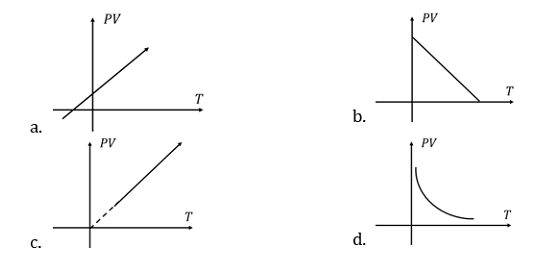PV = nRT ⇒ PV=CT

Therefore, PV v/s T graph is a straight line.

Question 13: In photoelectric effect stopping potential is 3V0 for incident wavelength λ0 and stopping potential for incident wavelength 2λ0. Find threshold wavelength.

a. 3λ
b. 2λ0
c. 4λ0
d. 8λ

KE = hv – W

eV = hc / λ – W

For first case

e(3Vo) = hc /λo – W ……..(i)

For second case:

e(Vo)=hc / 2λo – W ……(ii)

From equation (i) and (ii), we get,

W = hc / (4λo)

For λth

w = hc / λth

⇒ hc / (4λo) = hc/λth

⇒ λth = 4λo

Question 14: A plane electromagnetic wave travels in free space. Electric field is

$$\begin{array}{l}\left | \vec{E} \right |=E_{0}\hat{i}\end{array}$$
and magnetic field is represented by
$$\begin{array}{l}\left | \vec{B} \right |=B_{0}\hat{k}\end{array}$$
. What is the unit vector along the direction of propagation of electromagnetic waves?

a.

$$\begin{array}{l}\hat{j}\end{array}$$

b.
$$\begin{array}{l}-\hat{k}\end{array}$$

c.
$$\begin{array}{l}-\hat{j}\end{array}$$

d.
$$\begin{array}{l}\hat{k}\end{array}$$

Direction of the EM wave is given by direction of

$$\begin{array}{l}\vec{E}\times \vec{B}\end{array}$$
.

Unit vector in direction

$$\begin{array}{l}\vec{E}\times \vec{B}\end{array}$$
, =
$$\begin{array}{l}\frac{\vec{E}\times \vec{B}}{\left | \vec{E}\times \vec{B} \right |}\end{array}$$
$$\begin{array}{l}\frac{E_{o}\hat{i}\times B_{o}\hat{k}}{E_{o}B_{o}Sin\: 90^{o}}\end{array}$$
$$\begin{array}{l}\hat{i}\times \hat{k}\end{array}$$
$$\begin{array}{l}-\hat{J}\end{array}$$

Question 15: Two satellites of mass MA and MB are revolving around a planet of mass M in radius RA and RB respectively. Then

a. TA > TBif RA > R
b. TA> TB if MA> MB
c. TA= TB if MA> MB
d. TA > TB if RA< RB

According to Kepler’s law of planetary motion,

T ∝ R3/2

$$\begin{array}{l}\frac{T_{A}}{T_{B}} = \left ( \frac{R_{A}}{R_{B}} \right )^{\frac{3}{2}}\end{array}$$

So, if RA > RB then TA > TB.

Hence, option (a) is the correct answer.

Question 16: At 45° of the magnetic meridian angle of dip is 30° then find the angle of dip in a vertical plane at 45°?

a. tan−1(1/√6)
b. tan−1(1/√2)
c. tan−1 (1/√4)
d. tan−1(1/√3)

Let the horizontal and vertical components of the earth’s magnetic field at the meridian be V and H.

Angle of dip, tan⁡ θ = V / H……(i)

At angle of 45° from magnetic meridian, angle of dip = 30°

tan⁡ 30° = V / H cos⁡ 45°

⇒1 / √3 = V / H cos⁡ 45°

V / H = 1 / √6

tan ⁡θ = V / H = 1 / √6

⇒θ = tan-1 ⁡(1 / √6)

Question 17: A sodium lamp in space was emitting waves of wavelength 2880 Å. When observed from a planet, its wavelength was recorded at 2886 Å. Find the speed of the planet?

a. 4.25 × 105 m/s
b. 6.25 × 105 m/s
c. 2.75 × 105 m/s
d. 3.75 × 105 m/s

$$\begin{array}{l}\frac{V_{rel}}{C} = \frac{\Delta \lambda}{\lambda}\end{array}$$
$$\begin{array}{l}V_{rel} = \frac{6}{2880}\times3 \times 10^{8}\end{array}$$
$$\begin{array}{l}V_{rel} = 6.25 \times 10^{5} m/s\end{array}$$

Question 18: For a body in pure rolling, its rotational kinetic energy is 1/2 times of its translational kinetic energy. The body should be?

a. Solid cylinder
b. Ring
c. Solid sphere
d. Hollow sphere

Given,

Rotational K.E = ½ Translational K.E

½ Iω2 = ½ × ½ mv2

In pure rolling, v = Rω

½ Iω2 = ¼ mR2 ω2

I = ½ mR2

Hence, it is a solid cylinder.

Question 19: Magnetic susceptibility of a material is 499 & μ0 = 4π × 10−7 SI unit. Then find μr

a. 500
b. 400
c. 300
d. 200

Given, 𝜒 = 499

The relative permeability, μr = 1 + 𝜒 = 500

Question 20: An electron having the de Broglie wavelength λ falls on an X-ray tube. The cut-off wavelength of emitted X-ray is

a. 2mcλ2 / h
b. 2h / mc
c. h / mc
d. 2mcλ2 / 3h

De-broglie wavelength, λB = h / P

P = h / (λB)

Kinetic energy of electron, E = P2 / (2me) = h2 / 2me λB2

For cutoff wavelength of emitted X-ray: E = hc / λ

h2 / 2me λB2 = hc / λ

λ = 2me λB2 / h = 2mc λ2 / h where λB = λ and me = m

Question 21: A body is moved from rest along a straight line by a machine delivering constant power. Time taken by the body to travel a distance 𝑆 is proportional to:

a. 𝑆1/3
b. 𝑆2/3
c. 𝑆1/2
d. 𝑆1/4

Energy supplied in time t sec is,

E = P × t

Here P represents the power delivered by the machine.

⇒ Pt = ½ mv2

v ∝ √t

Writing the velocity in terms of the displacement of the body,

⇒ dS/dt = C√t

Here C is a constant.

$$\begin{array}{l}\int_{O}^{S}ds = C \int_{O}^{t} t^{\frac{1}{2}}dt\end{array}$$
$$\begin{array}{l}t^{\frac{3}{2}} = \frac{3S}{2C} \Rightarrow t = S^{\frac{2}{3}}\left ( \frac{3}{2C} \right ) \Rightarrow T\: \alpha \: S^{\frac{2}{3}}\end{array}$$

Question 22: A uniform rod of Young’s modulus Y is stretched by two tension forces T1 and T2 such that the rods get expanded to length L1 and L2 respectively. Find the initial length of the rod?

a.

$$\begin{array}{l}\frac{L_{1}T_{1}-L_{2}T_{2}}{T_{1}-T_{2}}\end{array}$$

b.
$$\begin{array}{l}\frac{L_{2}T_{1}-L_{1}T_{2}}{T_{2}-T_{1}}\end{array}$$

c.
$$\begin{array}{l}\frac{L_{1}T_{2}-L_{2}T_{2}}{T_{2}-T_{1}}\end{array}$$

d.
$$\begin{array}{l}\frac{L_{1}}{T_{1}}\times \frac{T_{2}}{L_{2}}\end{array}$$

Let the initial length of the rod to be Lo and area A

In this case the external force on the rod is tension hence from Hook’s law,

$$\begin{array}{l}\frac{F}{A} = Y\frac{\Delta l}{l}\end{array}$$

$$\begin{array}{l}\frac{T}{A} = Y\frac{\Delta l}{l}\end{array}$$

For both the cases we can relate the tension and elongation produced in rod as,

$$\begin{array}{l}\frac{T_{1}}{A} = \frac{Y(L_{1}-L_{o})}{L_{o}}\end{array}$$
……(i)

Here (L1 – Lo) is the elongation in rod when T1 is applied.

Similarly,

$$\begin{array}{l}\frac{T_{2}}{A} = \frac{Y(L_{2}-L_{o})}{L_{o}}\end{array}$$
……(ii)

Here (L2 – Lo) is the elongation in rod when T2 is applied.

On dividing Eq.(i) and (ii) we get,

$$\begin{array}{l}\frac{T_{1}}{T_{2}} = \frac{L_{1}-L_{o}}{L_{2}-L_{o}}\end{array}$$

⇒ T1 L2 – T1 Lo = T2 L1 – T2 Lo

Or,

$$\begin{array}{l}L_{o} = \frac{L_{1}T_{2}-L_{2}T_{1}}{T_{2}-T_{1}}\end{array}$$

Question 23: A block is projected up to a rough plane of inclination 30°. If time of ascending is half the time for descending and the coefficient of friction is μ =3/5√𝑛. Then 𝑛 =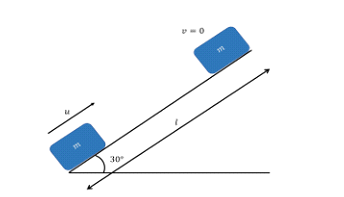S = ½ aAtA2 ….. (1)

S = ½ aDtD2 ….. (2)

From equation (1) and (2)

$$\begin{array}{l}\frac{t_{A}^{2}}{t_{D}^{2}} = \frac{a_{D}}{a_{A}}\end{array}$$

$$\begin{array}{l}\frac{t_{A}^{2}}{t_{D}^{2}} = \frac{g \: sin \: \Theta – \mu g \: cos \Theta}{g \: sin \: \Theta + \mu g \: cos \Theta}\end{array}$$

$$\begin{array}{l}\frac{t_{A}}{t_{D}} = \sqrt{\frac{g \: sin \: \Theta – \mu g \: cos \Theta}{g \: sin \: \Theta + \mu g \: cos \Theta}}\end{array}$$

$$\begin{array}{l}\frac{1}{2} = \sqrt{\frac{1-\sqrt{3\mu }}{1+\sqrt{3\mu }}}\end{array}$$

⇒ 1 + √3μ = 4 – 4√3 μ

⇒ 5√3μ = 3

⇒ μ = 3 / 5√3

Question 24: I-V characteristic curve of a diode in a forward bias is given in fig. Find out dynamic resistance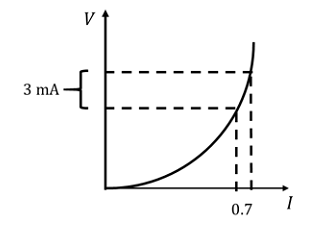a. 212.3 Ω
b. 205.3 Ω
c. 245.3 Ω
d. 233.3 Ω

Dynamic resistance = ΔV / ΔI

= 0.7 V / 3 mA = 233.3 Ω

Question 25: An electron is accelerated through a voltage of 40 kV. What will be its wavelength?

a. 0.061 Å
b. 0.011 Å
c. 0.021 Å
d. 0.161 Å

λB = h / P

= h / √2meV

=

$$\begin{array}{l}\frac{12.27}{\sqrt{V}}\end{array}$$
Å

=

$$\begin{array}{l}\frac{12.27}{\sqrt{40 \times 10^{3}}}\end{array}$$
= 0.061 Å

Question 26: Find the value of Rp in the given circuit? (VZ = 8V)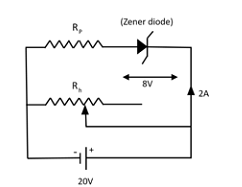a. 4Ω
b. 6Ω
c. 3Ω
d. 5Ω

Applying KVL

20 – 8 – 2RP = 0

RP = 6 Ω

Question 27: Two stars of masses m1 and m2 are in mutual interaction and revolving in orbits of radii r1 and r2 respectively. The time period of revolution for this system will be?

a.

$$\begin{array}{l}2\pi \sqrt{\frac{(r_{1}-r_{2})^{3}}{G(m_{1}+m_{2})}}\end{array}$$

b.
$$\begin{array}{l}2\pi \sqrt{\frac{(r_{1}+r_{2})^{3}}{G(m_{1}+m_{2})}}\end{array}$$

c.
$$\begin{array}{l}2\pi \sqrt{\frac{(r_{1}-r_{2})^{3}}{G(m_{1}-m_{2})}}\end{array}$$

d.
$$\begin{array}{l}2\pi \sqrt{\frac{(r_{1}+r_{2})^{3}}{G(m_{1}-m_{2})}}\end{array}$$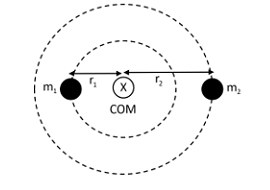Let angular velocity will be ω

For mass m1,

$$\begin{array}{l}\frac{Gm_{1}m_{2}}{(r_{1}+r_{2})^{2}} = m_{1}r_{1}\omega ^{2} = m_{1}\times \frac{m_{2}(r_{1}+r_{2})}{m_{1}+m_{2}}\omega ^{2}\end{array}$$
$$\begin{array}{l}\omega = \frac{\sqrt{G(m_{1}+m_{2})}}{(r_{1}+r_{2})^{\frac{3}{2}}}\end{array}$$
$$\begin{array}{l}T = \frac{2\pi }{\omega }\end{array}$$
$$\begin{array}{l}T = 2\pi\frac{(r_{1}+r_{2})^{3}}{G(m_{1}+m_{2})}\end{array}$$

Question 28: If N0 active nuclei become N0/16 in 80 days. Find half-life of nuclei?

a. 40 days
b. 20 days
c. 60 days
d. 30 days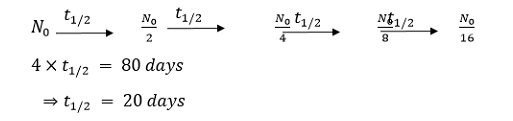Question 29: A gas is undergoing a change in state by an isothermal process AB as follow. Work done by gas in process AB is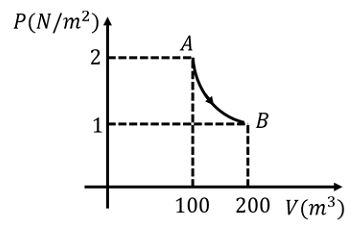a. 100 ln2 Joule
b. −100 ln2 Joule
c. 200 ln2 Joule
d. −200 ln2 Joule

Wisothermal = P1V1 ln⁡ V2 / V1

V1 = 100 m3

V2 = 200 m3

P1 = 2 N/m2

Wisothermal = 2 × 100 ln 200 / 100

W = 200 ln 2 Joule